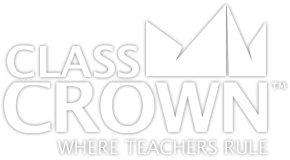Homeroom
Products
Store
Math Worksheets
Blog

# Fractions Worksheets5/5
Fractions Pack 1: Concepts
Skills included in this pack:
• Identifying prime and composite numbers
• Prime factorization
• Prime factorization with exponents
• Using divisibility rules
• Using divisibility rules in word problems
• Finding greatest common factor
• Identifying equivalent fractions
• Simplifying fractions
• Converting improper fractions into mixed numbers
• Converting mixed numbers into improper fractions
• Identifying fractions on a number line
• Comparing fractions
• Comparing decimals and fractions
• Converting between decimals and fractions
+ See More
Free Sample Pages Available5/5
Fractions Pack 2: Adding & Subtracting
Skills included in this pack:
• Adding fractions with like denominators
• Adding mixed numbers with like denominators, no regrouping
• Adding fractions with unlike denominators
• Subtracting fractions with like denominators
• Subtracting mixed numbers with like denominators
• Subtracting fractions with unlike denominators
• Adding mixed numbers with unlike denominators
• Subtracting mixed numbers from whole numbers
• Subtracting fractions with unlike denominators and with regrouping
• Adding and subtracting fractions word problems
• Adding and subtracting mixed numbers word problems
• Adding/subtracting fractions and mixed numbers
• Adding three or more fractions with unlike denominators
• Inequalities with adding and subtracting fractions
• Estimating sums of fractions
+ See More
Free Sample Pages Available5/5
Fractions Pack 3: Multiplying & Dividing
Skills included in this pack:
• Multiplying whole numbers by fractions
• Multiplying whole number by fractions, word problems
• Representing multiplication of fractions visually
• Multiplying two fractions
• Multiplying two fractions, word problems
• Multiplying three fractions
• Multiplying a mixed number by a whole number
• Multiplying mixed numbers and fractions
• Multiplying two mixed numbers
• Dividing whole numbers by fractions
• Dividing fractions by whole numbers
• Dividing two fractions
• Dividing fractions and mixed numbers
• Dividing two mixed numbers
• Multiplying and dividing fractions and mixed numbers, word problems
+ See More
Free Sample Pages AvailableSelf Checking

Our math worksheets introduce a puzzle aspect to math, giving students immediate feedback as to whether or not they are solving problems correctly. If the answer to the riddle isn't spelled correctly, the student knows which problems he's made an error on.Fun Puzzle AspectImmediate FeedbackProblem Solving Motivation

Each math worksheet contains a riddle that the student solves by completeing all the problems on the worksheet. This keeps kids motivated to complete each problem so that they can find the answer to the riddle.Common Core Aligned

All our math worksheet packs are designed with Common Core in mind. That way you don’t have to worry about whether your math ciriculum is aligned or not when you incorpoate ClassCrown Riddle-Me-Worksheets in your lesson plans.High Quality Design

Each page of our math worksheets has been produced in high resolution at 144 dpi and designed in full, vibrant color for maximum quality. They look stunning whether you are printing in color or black and white.High Resolution
(144 dpi)Stunning Color & Clarity